The area of quadrilateral is the region enclosed by sides of the quadrilateral. We have already acquainted with the term area. It is defined as the region occupied inside the boundary of a flat object or figure. The measurement is done in square units with the standard unit being square metres (m2). We know that the polygon with four sides is called a quadrilateral. A quadrilateral can be a square, rectangle, rhombus, kite, parallelogram and trapezium. In this article, we are going to learn the general formula for the area of a quadrilateral with many solved examples.

A quadrilateral is a polygon with four sides. A quadrilateral is a closed two-dimensional figure formed by joining the four points among which three points are non-collinear points. A quadrilateral has four sides, four angles and four vertices. The sides of the quadrilateral may or may not be equal.

• Every quadrilateral has 4 vertices and 4 sides enclosing 4 angles.
• The sum of its interior angles is 360 degrees.
• A quadrilateral, in general, has sides of different lengths and angles of different measures. However, squares, rectangles, parallelograms, etc. are special types of quadrilaterals with some of their sides and angles being equal.

The formula for the area of quadrilateral can be found using different methods such as dividing the quadrilateral into two triangles, or by using Heron’s formula or by using sides of the quadrilateral. Now let us discuss all these methods in detail.

### Area of Quadrilateral by Dividing it into Two Triangles

Consider a quadrilateral PQRS, of different (unequal) lengths, let us derive a formula for the area of a quadrilateral.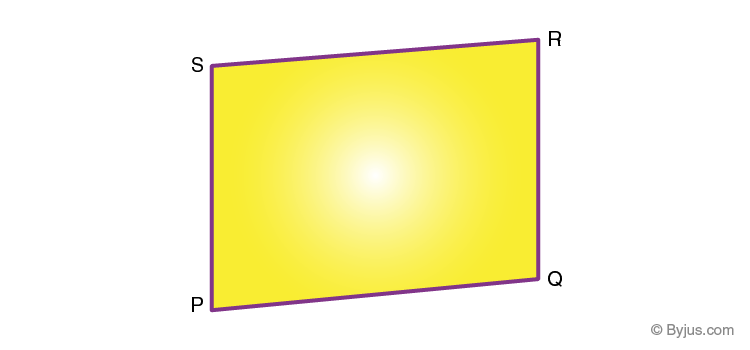• We can view the quadrilateral as a combination of 2 triangles, with the diagonal PR being the common base.
• h1 and h2 are the heights of triangles PSR and PQR, respectively.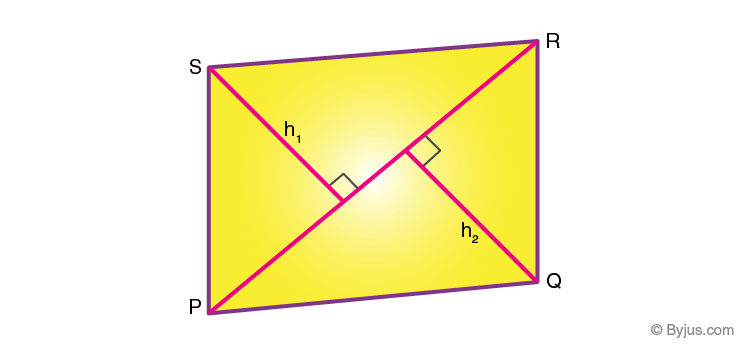• Area of quadrilateral PQRS is equal to the sum of the area of triangle PSR and the area of triangle PQR.
• Area of triangle PSR = (base * height)/2 = (PR * h1)/2
• Area of triangle PQR = (base * height)/2 = (PR* h2)/2
• Thus, area of quadrilateral PQRS is,
• Area of triangle PSR + Area of triangle PQR =
$$\begin{array}{l}\frac{PR \times h_{1}}{2} + \frac{PR \times h_{2}}{2} = PR \left ( \frac{h_{1}+ h_{2}}{2} \right )\end{array}$$
• $$\begin{array}{l}= \frac{1}{2} PR \times (h_{1}+ h_{2})\end{array}$$

Hence, the area of a quadrilateral formula is,

$$\begin{array}{l}Area\ of\ a\ general\ Quadrilateral= \frac{1}{2} \times \; diagonal \times (Sum \; of \; height \; of \; two \; triangle)\end{array}$$

### Area of Quadrilateral Using Heron’s Formula

We know that Heron’s formula is used to find the area of a triangle if three sides of the triangle are given. Follow the given procedure to find the area of the quadrilateral.

Step 1: Divide the quadrilateral into two triangles using a diagonal whose diagonal length is known.

Step 2: Now, apply Heron’s formula for each triangle to find the area of a quadrilateral.

[If a, b, c are the sides of a triangle, then Heron’s formula to find the area of a triangle is

Area of triangle = √[s(s-a)(s-b)(s-c)] square units

Where “s” is the semi-perimeter of triangle, which is equal to (a+b+c)/2. ]

Step 3: Now add the area of two triangles to get the area of a quadrilateral.

### Area of Quadrilateral Using Sides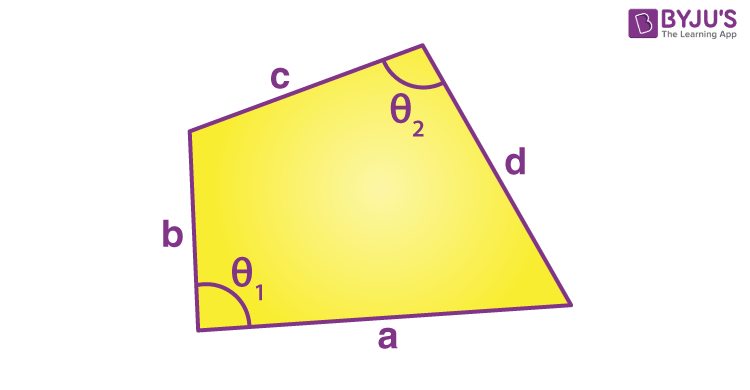If the sides of a quadrilateral (a, b, c, d) are given, and two of its opposite angles (θ1 and θ2) are given, then the area of quadrilateral can be calculated as follows:

$$\begin{array}{l}Area\ of\ Quadriateral = \sqrt{(s-a)(s-b)(s-c)(s-d)-abcd\ cos^{2}\frac{\theta }{2}} square\ units.\end{array}$$

Where “s” is the semi-perimeter of the quadrilateral.

(i.e.) s = (a+b+c+d)/2

And,  θ= θ12

### Area Formula for All Quadrilaterals

The area formulas for different types of quadrilaterals such as square, rectangle, rhombus, kite, parallelogram and trapezium are given below:

Area of Square = a2 square units.

Where “a” is the side length of a square.

Area of Rectangle = l×b square units

Where “l” and “b” are the length and breadth of a rectangle respectively.

Area of Parallelogram = b×h square units

Where “b” and “h” are the base length and height of the parallelogram.

Area of Rhombus = (½)×d1×d2 square units.

Where “d1” and “d2” are the two diagonals of the rhombus.

Area of kite = (½)pq square units.

Where “p” and “q” are the two diagonals of the kite.

Area of Trapezium = (½)(a+b)h square units.

Where “a” and “b” are the side lengths of parallel sides and “h” is the height of the trapezium.

Let us have a look at the table given below for different types of quadrilaterals and the formulas to find the area of each quadrilateral as mentioned above along with the corresponding shapes.

 Quadrilateral Name Shape Area formula Square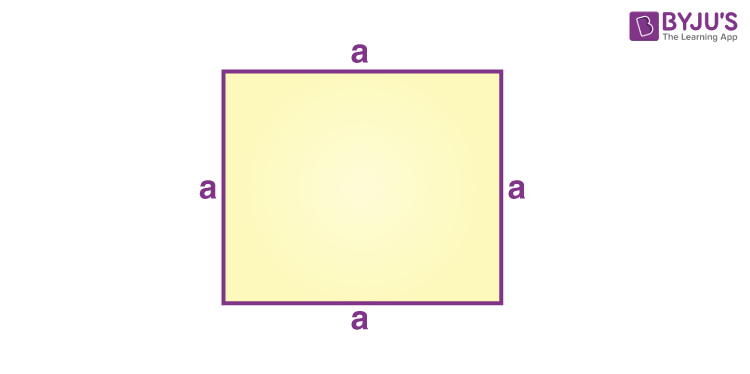a × a = a2 Rectangle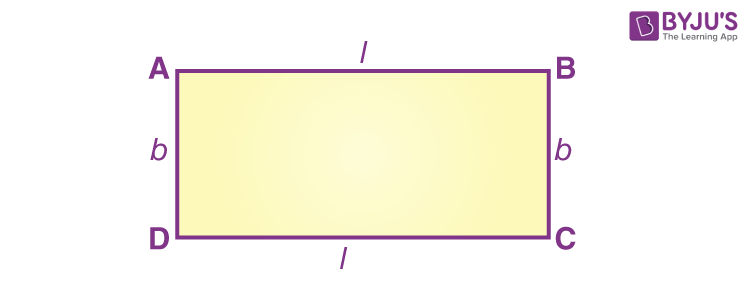lb Parallelogram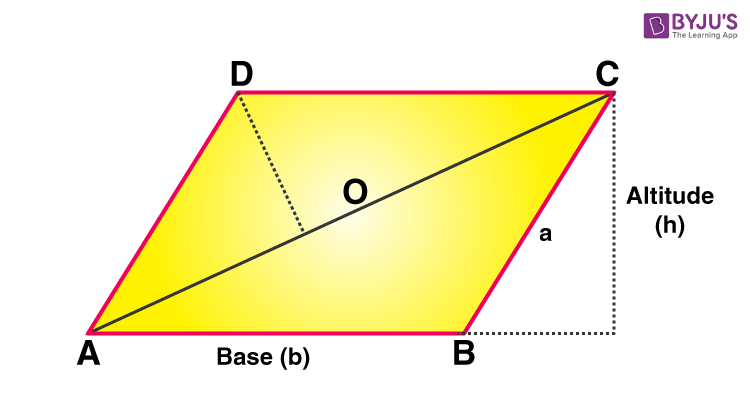b × h Rhombus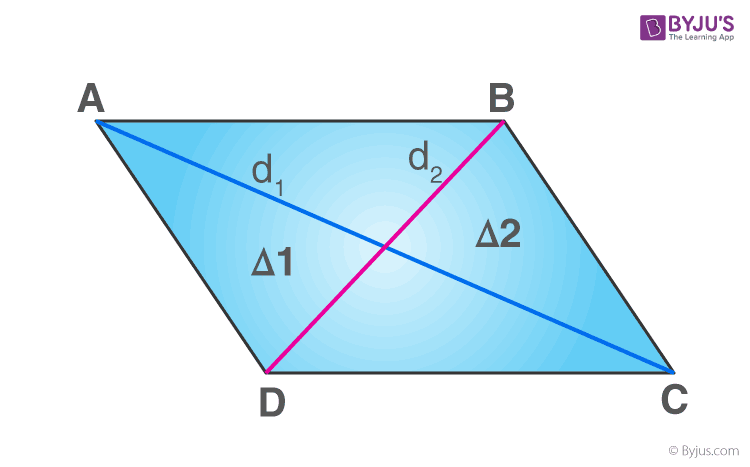(½) × d1 × d2 Kite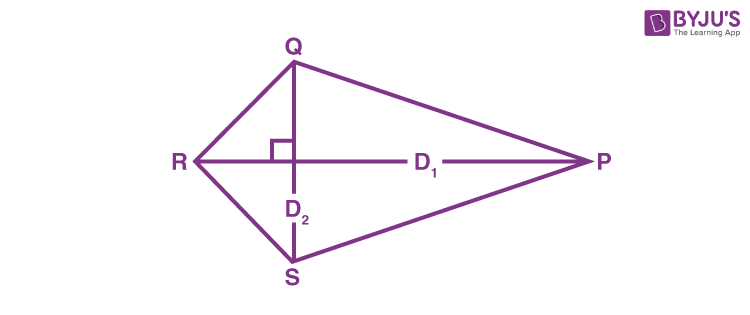(½) × D1 × D2 Trapezium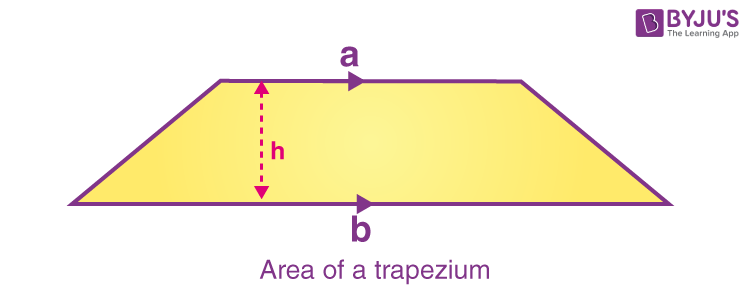(½) × (a + b) × h

### Area of a Quadrilateral Examples

Example 1:

In the given quadrilateral ABCD, the side BD = 15 cm and the heights of the triangles ABD and BCD are 5 cm and 7 cm, respectively. Find the area of the quadrilateral ABCD.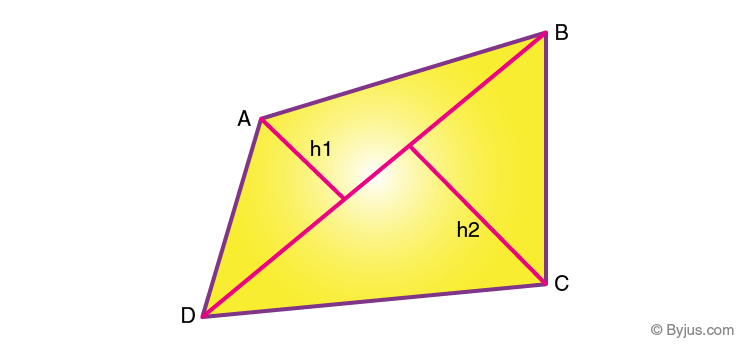Solution:

Diagonal = BD = 15 cm

Heights, h1= 5 cm & h2 = 7 cm

Sum of the heights of the triangles = h1 + h2 = 5 + 7 = 12 cm

Thus, area of quadrilateral ABCD is:

$$\begin{array}{l}A= \frac{1}{2} \times \; diagonal \times (Sum \; of \; height \; of \; two \; triangle)\end{array}$$

= (15 * 12)/2 = 90 cm2

Example 2:

Find the area of a rhombus whose diagonals are 7 cm and 6 cm respectively.

Solution:

Given: Diagonal 1, p = 7 cm

Diagonal 2, q = 6 cm.

The area of rhombus = (½)×d1×d2 square units.

A = (½)(7)(6) cm2

A = 7(3) cm2

A = 21 cm2.

Therefore, the area of a rhombus is 21 cm2.

## Area of Quadrilateral with Vertices

In coordinate geometry, the area of the quadrilateral can be calculated using the vertices quadrilateral.

Let A(x1, y1), B(x2, y2), C(x3, y3) and D(x4, y4) be the vertices of a quadrilateral ABCD.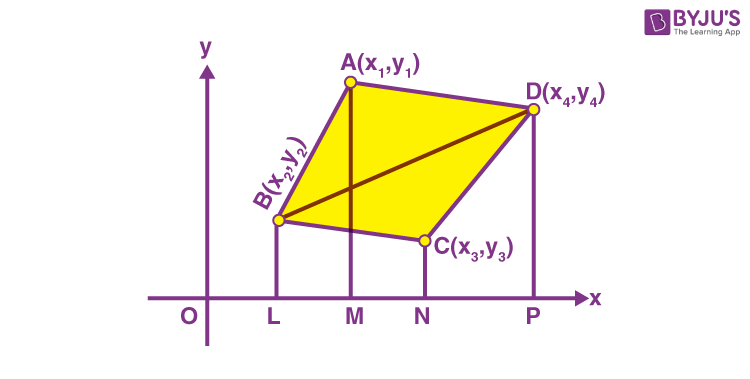Here, we can find the area of the quadrilateral in two ways.

Method 1:

To find the area of the quadrilateral ABCD, we have to choose the vertices A(x1, y1), B(x2, y2), C(x3, y3) and D(x4, y4) of the quadrilateral ABCD in order (counterclockwise direction) and write them column-wise as shown below.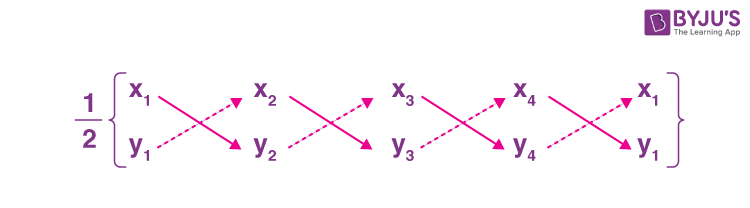Observe the directions given in the dark arrows, add the diagonal products, i.e., x1y2, x2y3, x3y4 and x4y1.

(x1y2 + x2y3 + x3y4 + x4y1)….(i)

Now, consider the dotted arrows and add the diagonal products, i.e., x2y1, x3y2, x4y3 and x1y4.

(x2y1 + x3y2 + x4y3 + x1y4)….(ii)

Subtract (ii) from (i) and multiply the difference by 1/2.

(1/2) ⋅ [(x1y2 + x2y3 + x3y4 + x4y1) – (x2y1 + x3y2 + x4y3 + x1y4)]

Therefore, the formula for the area of quadrilateral using vertices is:

A =(1/2) ⋅ [(x1y2 + x2y3 + x3y4 + x4y1) – (x2y1 + x3y2 + x4y3 + x1y4)]

Method 2:

In this method, we need to divide the given quadrilateral into two triangles. Then, find the area of each triangle and add it up to get the area of the quadrilateral.

Area of quadrilateral ABCD = Area of triangle ABD + Area of triangle BCD

Note:

Area of triangke with vertices P(x1, y1), Q(x2, y2) and R(x3, y3) is given by:

(1/2) |x1(y2 – y3) + x2(y3 – y1) + x3(y1 – y2)|

Example: Calculate the area of the quadrilateral formed with the vertices (−3, 2), (5, 4), (7, −6) and (−5, −4).

Solution:

Let A(-3, 2), B(5, 4), C(7, -6) and D(-5, -4) be the vertices of a quadrilateral ABCD.

Thus,

A(-3, 2) = (x1, y1)

B(5, 4) = (x2, y2

C(7, -6) = (x3, y3)

D(-5, -4) = (x4, y4)

We know that,

Area of quadrilateral ABCD = (1/2) ⋅ [(x1y2 + x2y3 + x3y4 + x4y1) – (x2y1 + x3y2 + x4y3 + x1y4)]

Substituting the values,

= (½). {[-3(4) + 5(-6) + 7(-4) + (-5)2] – {[5(2) + 7(4) + (-5)(-6) + (-3)(-4)]}

= (½).[(-12 – 30 – 28 – 10) – (10 + 28 + 30 + 12)]

= (½) [-80 – 80]

= 160/2 {since area cannot be negative}

= 80

Therefore, the area of the quadrilateral formed with the given vertices is 80 sq. units.

### Practice Problems

1. Find the area of a rectangle whose length is 12 cm and breadth is 9 cm.
2. What is the area of a trapezium whose parallel sides measure 13 cm, 8 cm and the distance between these parallel sides is 10 cm?
3. Find the area of the quadrilateral whose vertices are (8, 6), (5, 11), (-5, 12) and (-4, 3).

Q1

### What is the area of a quadrilateral?

The area of the quadrilateral is the space occupied by the shape quadrilateral in the two-dimensional space. As we know, a quadrilateral is a 2D figure with four sides. Generally, a quadrilateral is the combined form of a regular or an irregular triangle.

Q2

### Mention the different types of quadrilateral.

The different types of a quadrilateral are:
Square
Rectangle
Rhombus
Kite
Parallelogram
Trapezium

Q3

### How to calculate the area of a quadrilateral?

The quadrilateral is the combination of the basic geometric shape called triangles. To calculate the area of a quadrilateral, the area of the individual triangles should be computed, and add the area of the individual triangles.

Q4

### Mention the applications of quadrilaterals.

Quadrilaterals and its area are mostly used in the field of architecture, agriculture, designing, and navigation to find the actual distance with precision.

Q5

### How to calculate the area of a quadrilateral if one of its diagonals and perpendiculars from the vertices are given?

If the diagonal and the length of the perpendiculars from the vertices are given, then the area of the quadrilateral is calculated as:
Area of quadrilateral = (½) × diagonal length × sum of the length of the perpendiculars drawn from the remaining two vertices.

Stay tuned with BYJU’S – The Learning App to learn more on the area of different quadrilaterals quickly by watching the personalized videos.